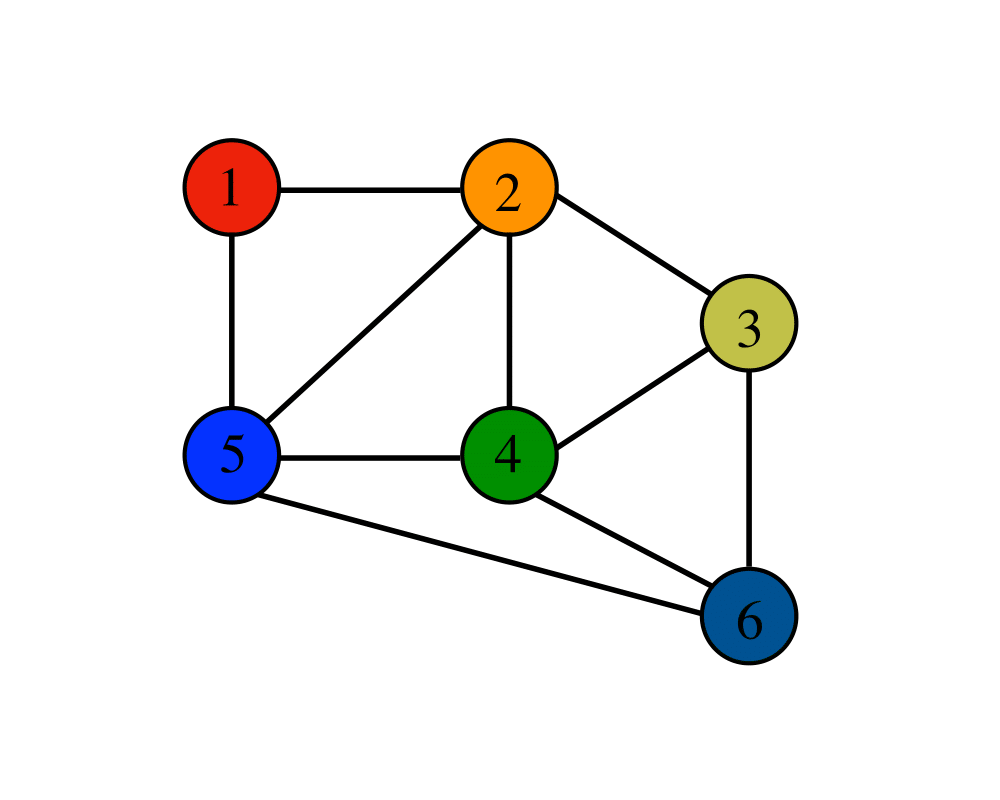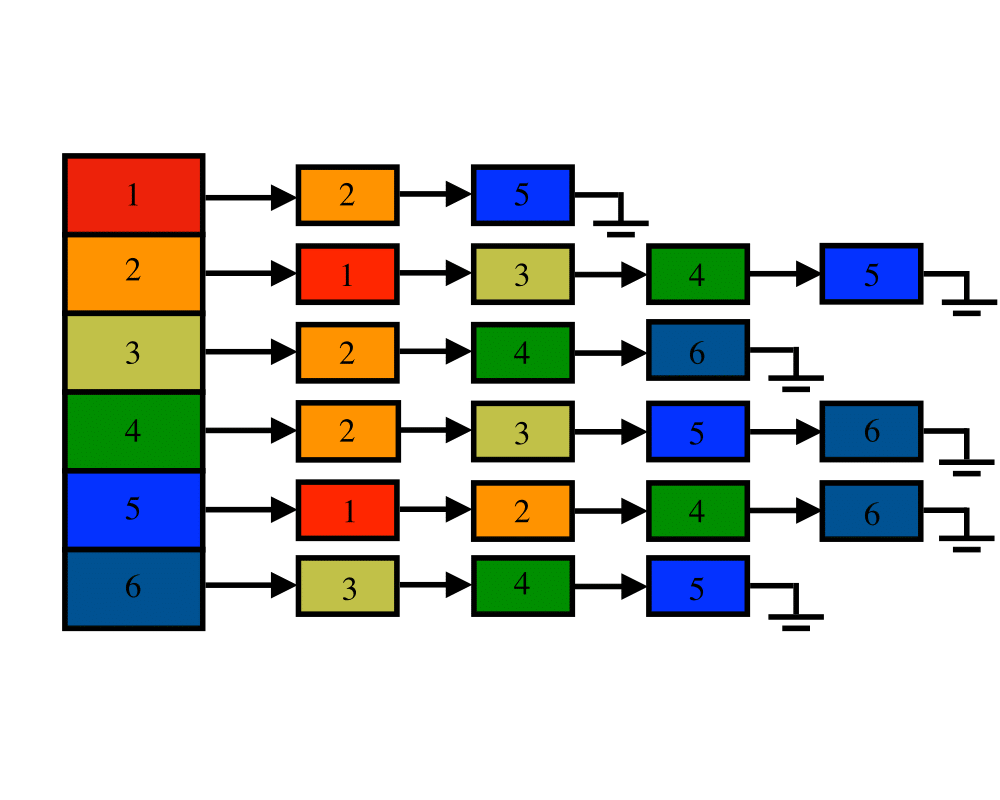# Graph Data StructuresInputOutput

Input Description: A graph $$G$$.
Problem: Give an efficient, flexible data structure to represent $$G$$.

Excerpt from The Algorithm Design Manual: While there are several possible variations, the two basic data structures for graphs are adjacency matrices and adjacency lists.

• How dense will your graph be? If the graph is very dense, meaning that a large fraction of the vertex pairs define edges, there is probably no compelling reason to use adjacency lists, since you will be doomed to using Theta(n2) space, anyway.
• Which algorithms will you be implementing? Certain algorithms are easier on adjacency matrices (such as all-pairs shortest path) and others on adjacency lists (such as most DFS-based algorithms). Adjacency matrices win for algorithms that repeatedly ask, Is (i,j) in G?'' However, most graph algorithms can be modified to eliminate such queries.
• Will you be modifying the graph over the course of your application, and if so, how? Repeated edge insertions and (particularly) deletions argue for adjacency matrices, or perhaps for fancier versions of adjacency lists such as binary search trees. However, more likely than modifying the topology of graph is modifying the attributes of a vertex or edge of the graph, such as size, weight, or color. Attributes are best handled as extra fields in the vertex or edge records of adjacency lists.

Building a good general-purpose graph type is surprisingly tricky and difficult. For this reason, we suggest that you check out existing implementations (particularly LEDA) before hacking up your own. Note that it costs only time linear in the size of the larger data structure to convert between adjacency matrices and adjacency lists. This conversion is unlikely to be the bottleneck in any application, if you decide you want to use both data structures and have the space to store them. This usually isn't necessary but might prove simplest if you are confused about the alternatives.

Go To Main Page